JMSLTM Numerical Library 7.2.0
com.imsl.math

## Class SuperLU

• All Implemented Interfaces:
Serializable

```public class SuperLU
extends Object
implements Serializable```
Computes the LU factorization of a general sparse matrix of type `SparseMatrix` by a column method and solves the real sparse linear system of equations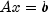.

Consider the sparse linear system of equations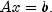Here, A is a general square, nonsingular, n by n sparse matrix, and x and b are vectors of length n. All entries in A, x and b are of type `double`.

Gaussian elimination, applied to the system above, can be shortly described as follows:
1. Compute a triangular factorization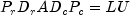. Here,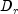and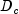are positive definite diagonal matrices to equilibrate the system and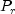and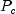are permutation matrices to ensure numerical stability and preserve sparsity. L is a unit lower triangular matrix and U is an upper triangular matrix.

2. Solveby evaluating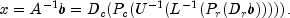This is done efficiently by multiplying from right to left in the last expression: Scale the rows of b by. Multiplying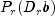means permuting the rows of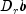. Multiplying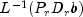means solving the triangular system of equations with matrix L by substitution. Similarly, multiplying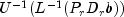means solving the triangular system with U.

Class `SuperLU` handles step 1 above in the `solve` method if it has not been computed prior to step 2. More precisely, beforeis solved the following steps are performed:

1. Equilibrate matrix A, i.e. compute diagonal matricesandso that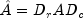is "better conditioned" than A, i.e.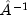is less sensitive to perturbations in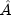than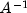is to perturbations in A.
2. Order the columns ofto increase the sparsity of the computed L and U factors, i.e. replaceby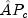whereis a column permutation matrix.
3. Compute the LU factorization of. For numerical stability, the rows ofare eventually permuted through the factorization process by scaled partial pivoting, leading to the decomposition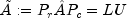. The LU factorization is done by a left looking supernode-panel algorithm with 2-D blocking. See Demmel, Eisenstat, Gilbert et al. (1999) for further information on this technique.
4. Compute the reciprocal pivot growth factor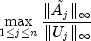where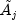and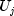denote the j-th column of matrices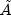and U, respectively.
5. Estimate the reciprocal of the condition number of matrix.
Method `solve` uses this information to perform the following steps:
1. Solve the systemusing the computed triangular factors.
2. Iteratively refine the solution, again using the computed triangular factors. This is equivalent to Newton's method.
3. Compute forward and backward error bounds for the solution vector x.

Some of the steps mentioned above are optional. Their settings can be controlled by the set methods of class `SuperLU`.

Class `SuperLU` is based on the SuperLU code written by Demmel, Gilbert, Li et al. For more detailed explanations of the factorization and solve steps, see the SuperLU Users' Guide (1999).

Copyright (c) 2003, The Regents of the University of California, through Lawrence Berkeley National Laboratory (subject to receipt of any required approvals from U.S. Dept. of Energy)

Redistribution and use in source and binary forms, with or without modification, are permitted provided that the following conditions are met:

(1) Redistributions of source code must retain the above copyright notice, this list of conditions and the following disclaimer.

(2) Redistributions in binary form must reproduce the above copyright notice, this list of conditions and the following disclaimer in the documentation and/or other materials provided with the distribution.

(3) Neither the name of Lawrence Berkeley National Laboratory, U.S. Dept. of Energy nor the names of its contributors may be used to endorse or promote products derived from this software without specific prior written permission.

THIS SOFTWARE IS PROVIDED BY THE COPYRIGHT HOLDERS AND CONTRIBUTORS "AS IS" AND ANY EXPRESS OR IMPLIED WARRANTIES, INCLUDING, BUT NOT LIMITED TO, THE IMPLIED WARRANTIES OF MERCHANTABILITY AND FITNESS FOR A PARTICULAR PURPOSE ARE DISCLAIMED. IN NO EVENT SHALL THE COPYRIGHT OWNER OR CONTRIBUTORS BE LIABLE FOR ANY DIRECT, INDIRECT, INCIDENTAL, SPECIAL, EXEMPLARY, OR CONSEQUENTIAL DAMAGES (INCLUDING, BUT NOT LIMITED TO, PROCUREMENT OF SUBSTITUTE GOODS OR SERVICES; LOSS OF USE, DATA, OR PROFITS; OR BUSINESS INTERRUPTION) HOWEVER CAUSED AND ON ANY THEORY OF LIABILITY, WHETHER IN CONTRACT, STRICT LIABILITY, OR TORT (INCLUDING NEGLIGENCE OR OTHERWISE) ARISING IN ANY WAY OUT OF THE USE OF THIS SOFTWARE, EVEN IF ADVISED OF THE POSSIBILITY OF SUCH DAMAGE.

Example, Serialized Form
• ### Field Summary

Fields
Modifier and Type Field and Description
`static int` `COLUMN_APPROXIMATE_MINIMUM_DEGREE`
For column ordering, use column approximate minimum degree ordering.
`static int` `COLUMN_SCALING`
Indicates that input matrix A was column scaled before factorization.
`static int` `FILL_FACTOR`
A performance tuning parameter which can be adjusted via method `setPerformanceTuningParameters`.
`static int` `MAXIMUM_SUPERNODE_SIZE`
A performance tuning parameter which can be adjusted via method `setPerformanceTuningParameters`.
`static int` `MINIMUM_COLUMN_DIMENSION`
A performance tuning parameter which can be adjusted via method `setPerformanceTuningParameters`.
`static int` `MINIMUM_DEGREE_AT_A`
For column ordering, use minimum degree ordering on the structure of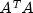.
`static int` `MINIMUM_DEGREE_AT_PLUS_A`
For column ordering, use minimum degree ordering on the structure of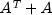.
`static int` `MINIMUM_ROW_DIMENSION`
A performance tuning parameter which can be adjusted via method `setPerformanceTuningParameters`.
`static int` `NATURAL_ORDERING`
For column ordering, use the natural ordering.
`static int` `NO_SCALING`
Indicates that input matrix A was not equilibrated before factorization.
`static int` `PANEL_SIZE`
A performance tuning parameter which can be adjusted via method `setPerformanceTuningParameters`.
`static int` `RELAXATION_PARAMETER`
A performance tuning parameter which can be adjusted via method `setPerformanceTuningParameters`.
`static int` `ROW_AND_COLUMN_SCALING`
Indicates that input matrix A was row and column scaled before factorization.
`static int` `ROW_SCALING`
Indicates that input matrix A was row scaled before factorization.
• ### Constructor Summary

Constructors
Constructor and Description
`SuperLU(SparseMatrix A)`
Constructor for `SuperLU`.
• ### Method Summary

Methods
Modifier and Type Method and Description
`int` `getColumnPermutationMethod()`
Returns the method that will be used to permute the columns of the input matrix.
`double` `getConditionNumber()`
Returns the estimate of the reciprocal condition number of the matrix A.
`double` `getDiagonalPivotThreshold()`
Returns the threshold used for a diagonal entry to be an acceptable pivot.
`boolean` `getEquilibrate()`
Returns the equilibration flag.
`int` `getEquilibrationMethod()`
Returns information on the type of equilibration used before matrix factorization.
`double` `getForwardErrorBound()`
Returns the estimated forward error bound for the solution vector.
`boolean` `getIterativeRefinement()`
Returns a value specifying whether iterative refinement is to be performed or not.
`int` `getPerformanceTuningParameters(int parameter)`
Returns a performance tuning parameter value.
`boolean` `getPivotGrowth()`
Returns the reciprocal pivot growth factor flag.
`double` `getReciprocalPivotGrowthFactor()`
Returns the reciprocal pivot growth factor.
`double` `getRelativeBackwardError()`
Returns the componentwise relative backward error of the solution vector.
`boolean` `getSymmetricMode()`
Returns the symmetric mode flag.
`void` `setColumnPermutationMethod(int colpermute)`
Specifies how to permute the columns of the input matrix.
`void` `setDiagonalPivotThreshold(double thresh)`
Specifies the threshold used for a diagonal entry to be an acceptable pivot.
`void` `setEquilibrate(boolean equilibrate)`
Determines if input matrix A should be equilibrated before factorization.
`void` `setIterativeRefinement(boolean refine)`
Specifies whether to perform iterative refinement.
`void` ```setPerformanceTuningParameters(int parameter, int tunedValue)```
Sets performance tuning parameters.
`void` `setPivotGrowth(boolean growth)`
Specifies whether to compute the reciprocal pivot growth factor.
`void` `setSymmetricMode(boolean symmetric)`
Specifies whether to use the symmetric mode.
`double[]` `solve(double[] b)`
Computation of the solution vector for the system.
`double[]` `solveTranspose(double[] b)`
Computation of the solution vector for the system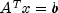.
• ### Methods inherited from class java.lang.Object

`clone, equals, finalize, getClass, hashCode, notify, notifyAll, toString, wait, wait, wait`
• ### Field Detail

• #### COLUMN_APPROXIMATE_MINIMUM_DEGREE

`public static final int COLUMN_APPROXIMATE_MINIMUM_DEGREE`
For column ordering, use column approximate minimum degree ordering.
Constant Field Values
• #### COLUMN_SCALING

`public static final int COLUMN_SCALING`
Indicates that input matrix A was column scaled before factorization. This is a return value for `getEquilibrationMethod`.
Constant Field Values
• #### FILL_FACTOR

`public static final int FILL_FACTOR`
A performance tuning parameter which can be adjusted via method `setPerformanceTuningParameters`.
Constant Field Values
• #### MAXIMUM_SUPERNODE_SIZE

`public static final int MAXIMUM_SUPERNODE_SIZE`
A performance tuning parameter which can be adjusted via method `setPerformanceTuningParameters`.
Constant Field Values
• #### MINIMUM_COLUMN_DIMENSION

`public static final int MINIMUM_COLUMN_DIMENSION`
A performance tuning parameter which can be adjusted via method `setPerformanceTuningParameters`.
Constant Field Values
• #### MINIMUM_DEGREE_AT_A

`public static final int MINIMUM_DEGREE_AT_A`
For column ordering, use minimum degree ordering on the structure of.
Constant Field Values
• #### MINIMUM_DEGREE_AT_PLUS_A

`public static final int MINIMUM_DEGREE_AT_PLUS_A`
For column ordering, use minimum degree ordering on the structure of.
Constant Field Values
• #### MINIMUM_ROW_DIMENSION

`public static final int MINIMUM_ROW_DIMENSION`
A performance tuning parameter which can be adjusted via method `setPerformanceTuningParameters`.
Constant Field Values
• #### NATURAL_ORDERING

`public static final int NATURAL_ORDERING`
For column ordering, use the natural ordering.
Constant Field Values
• #### NO_SCALING

`public static final int NO_SCALING`
Indicates that input matrix A was not equilibrated before factorization. This is a return value for `getEquilibrationMethod`.
Constant Field Values
• #### PANEL_SIZE

`public static final int PANEL_SIZE`
A performance tuning parameter which can be adjusted via method `setPerformanceTuningParameters`.
Constant Field Values
• #### RELAXATION_PARAMETER

`public static final int RELAXATION_PARAMETER`
A performance tuning parameter which can be adjusted via method `setPerformanceTuningParameters`.
Constant Field Values
• #### ROW_AND_COLUMN_SCALING

`public static final int ROW_AND_COLUMN_SCALING`
Indicates that input matrix A was row and column scaled before factorization. This is a return value for `getEquilibrationMethod`.
Constant Field Values
• #### ROW_SCALING

`public static final int ROW_SCALING`
Indicates that input matrix A was row scaled before factorization. This is a return value for `getEquilibrationMethod`.
Constant Field Values
• ### Constructor Detail

• #### SuperLU

`public SuperLU(SparseMatrix A)`
Constructor for `SuperLU`.
Parameters:
`A` - a `SparseMatrix` containing the sparse quadratic input matrix.
• ### Method Detail

• #### getColumnPermutationMethod

`public int getColumnPermutationMethod()`
Returns the method that will be used to permute the columns of the input matrix.
Returns:
an `int` scalar specifying how the columns of the input matrix are to be permuted for sparsity preservation.  return value method 0 = `NATURAL_ORDERING` natural ordering, that is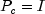, I the identity matrix. 1 = `MINIMUM_DEGREE_AT_PLUS_A` minimum degree ordering on the structure of2 = `MINIMUM_DEGREE_AT_A` minimum degree ordering on the structure of3 = `COLUMN_APPROXIMATE_MINIMUM_DEGREE` column approximate minimum degree ordering
• #### getConditionNumber

```public double getConditionNumber()
throws SingularMatrixException```
Returns the estimate of the reciprocal condition number of the matrix A.
Returns:
a `double` scalar containing the reciprocal condition number of the matrix A after equilibration and permutation of rows/columns (if done). If the return value is less than machine precision (in particular, if the return value` = 0`), the matrix is singular to working precision.
Throws:
`SingularMatrixException`
• #### getDiagonalPivotThreshold

`public double getDiagonalPivotThreshold()`
Returns the threshold used for a diagonal entry to be an acceptable pivot.
Returns:
a `double` scalar specifying the threshold used for a diagonal entry to be an acceptable pivot.
• #### getEquilibrate

`public boolean getEquilibrate()`
Returns the equilibration flag.
Returns:
a `boolean` specifying whether or not matrix `A` is equilibrated before factorization. if ```getEquilibrate ``` returns `true` the system is equilibrated, if `getEquilibrate` returns `false`, no equilibration is performed.
• #### getEquilibrationMethod

`public int getEquilibrationMethod()`
Returns information on the type of equilibration used before matrix factorization.
Returns:
an `int` value specifying the equilibration option used.  return value Description 1 = `NO_SCALING` No equilibration is performed. 2 = `ROW_SCALING` Equilibration is performed with row scaling. 3 = `COLUMN_SCALING` Equilibration is performed with column scaling. 4 = `ROW_AND_COLUMN_SCALING` Equilibration is performed with row and column scaling.
• #### getForwardErrorBound

`public double getForwardErrorBound()`
Returns the estimated forward error bound for the solution vector.
Returns:
a `double` containing the estimated forward error bound for the solution vector. The estimate is as reliable as the estimate for the reciprocal condition number, and is almost always a slight overestimate of the true error. If iterative refinement is not used, the return value ` = 1.0`.
• #### getIterativeRefinement

`public boolean getIterativeRefinement()`
Returns a value specifying whether iterative refinement is to be performed or not.
Returns:
a `boolean` scalar specifying whether iterative refinement is to be performed, `true`, or no iterative refinement is to be performed, `false`.
• #### getPerformanceTuningParameters

`public int getPerformanceTuningParameters(int parameter)`
Returns a performance tuning parameter value.
Parameters:
`parameter` - an `int` scalar that specifies the parameter for which the value is to be returned.  parameter return value description `PANEL_SIZE` The panel size. `RELAXATION_PARAMETER` The relaxation parameter to control supernode amalgamation. `MAXIMUM_SUPERNODE_SIZE` The maximum allowable size for a supernode. `MINIMUM_ROW_DIMENSIONM` The minimum row dimension to be used for 2D blocking. `MINIMUM_COLUMN_DIMENSION` The minimum column dimension to be used for 2D blocking. `FILL_FACTOR` The estimated fill factor for L and U, compared with A.

Returns:
an `int` specifying the current value used for the specified tuning parameter.
• #### getPivotGrowth

`public boolean getPivotGrowth()`
Returns the reciprocal pivot growth factor flag.
Returns:
a `boolean` specifying whether to compute the reciprocal pivot growth factor. Returns true if the reciprocal pivot growth factor is to be computed.
• #### getReciprocalPivotGrowthFactor

```public double getReciprocalPivotGrowthFactor()
throws SingularMatrixException```
Returns the reciprocal pivot growth factor.
Returns:
a `double` scalar representing the reciprocal growth factor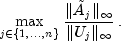If the returned value is much less than 1, the stability of the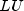factorization could be poor.
Throws:
`SingularMatrixException`
• #### getRelativeBackwardError

`public double getRelativeBackwardError()`
Returns the componentwise relative backward error of the solution vector.
Returns:
a `double` containing the componentwise relative backward error of the solution vector `x`. If iterative refinement is not used, the return value ` = 1.0`.
• #### getSymmetricMode

`public boolean getSymmetricMode()`
Returns the symmetric mode flag.
Returns:
a `boolean` scalar indicating if symmetric mode is to be used. Returns true if symmetric mode is to be used.
• #### setColumnPermutationMethod

`public void setColumnPermutationMethod(int colpermute)`
Specifies how to permute the columns of the input matrix.
Parameters:
`colpermute` - an `int` scalar specifying how to permute the columns of the input matrix for sparsity preservation.  colpermute method `NATURAL_ORDERING` natural ordering, that is, I the identity matrix. `MINIMUM_DEGREE_AT_PLUS_A` minimum degree ordering on the structure of`MINIMUM_DEGREE_AT_A` minimum degree ordering on the structure of`COLUMN_APPROXIMATE_MINIMUM_DEGREE` column approximate minimum degree ordering

If this method is not called, `colpermute` is set to `SuperLU.COLUMN_APPROXIMATE_MINIMUM_DEGREE`.
Throws:
`IllegalArgumentException` - is thrown when `colpermute` is not one of the above values.
• #### setDiagonalPivotThreshold

`public void setDiagonalPivotThreshold(double thresh)`
Specifies the threshold used for a diagonal entry to be an acceptable pivot.
Parameters:
`thresh` - a `double` scalar specifying the threshold used for a diagonal entry to be an acceptable pivot.
Default: `thresh=1.0`, i.e. classical partial pivoting.
Throws:
`IllegalArgumentException` - is thrown when `thresh` is not in the interval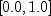.
• #### setEquilibrate

`public void setEquilibrate(boolean equilibrate)`
Determines if input matrix A should be equilibrated before factorization.
Parameters:
`equilibrate` - a `boolean` determining if matrix A should be equilibrated before the factorization.  equilibrate action `false` do not equilibrate `true` equilibrate
If this method is not called, `equilibrate` is set to true.
• #### setIterativeRefinement

`public void setIterativeRefinement(boolean refine)`
Specifies whether to perform iterative refinement.
Parameters:
`refine` - a `boolean` specifying whether to use iterative refinement, `refine = true`, or no iterative refinement, `refine = false`.
Default: `refine = false`.
• #### setPerformanceTuningParameters

```public void setPerformanceTuningParameters(int parameter,
int tunedValue)```
Sets performance tuning parameters.
Parameters:
`parameter` - an `int` scalar that specifies the parameter to be tuned.
`tunedValue` - an `int` scalar that specifies the value to be used for the specified tuning parameter.  parameter Description Default `PANEL_SIZE` The panel size. 10 `RELAXATION_PARAMETER` The relaxation parameter to control supernode amalgamation. 5 `MAXIMUM_SUPERNODE_SIZE` The maximum allowable size for a supernode. 100 `MINIMUM_ROW_DIMENSION` The minimum row dimension to be used for 2D blocking. 200 `MINIMUM_COLUMN_DIMENSION` The minimum column dimension to be used for 2D blocking. 40 `FILL_FACTOR` The estimated fill factor for L and U, compared with A. 20

Throws:
`IllegalArgumentException` - is thrown when a) `parameter` is not in the interval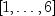or b) `tunedValue` is not greater than zero.
• #### setPivotGrowth

`public void setPivotGrowth(boolean growth)`
Specifies whether to compute the reciprocal pivot growth factor.
Parameters:
`growth` - a `boolean` specifying whether to compute the reciprocal pivot growth factor.  growth action `false` don't compute growth factor `true` compute growth factor
Default: `growth = false`.
• #### setSymmetricMode

`public void setSymmetricMode(boolean symmetric)`
Specifies whether to use the symmetric mode.
Parameters:
`symmetric` - a `boolean` indicating if symmetric mode is to be used. This mode should be applied if the input matrix A is diagonally dominant or nearly so. The user should then define a small diagonal pivot threshold (e.g. 0.0 or 0.01) by method `setDiagonalPivotThreshold` and choose an ()-based column permutation algorithm (e.g. column permutation method `SuperLU.MINIMUM_DEGREE_AT_PLUS_A`).  symmetric action `false` symmetric mode is not used `true` symmetric mode is used
Default: `symmetric=false`.
• #### solve

```public double[] solve(double[] b)
throws SingularMatrixException```
Computation of the solution vector for the system.
Parameters:
`b` - a `double` vector of length `n`, `n` the order of input matrix `A`, containing the right hand side.
Returns:
a `double` vector containing the solution to the system. Optionally, the solution is improved by iterative refinement, if `setIterativeRefinement` is set to `true`. Method `solve` internally first factorizes matrix A (step 1 of the introduction) if the factorization has not been done before.
Throws:
`SingularMatrixException`
• #### solveTranspose

```public double[] solveTranspose(double[] b)
throws SingularMatrixException```
Computation of the solution vector for the system.
Parameters:
`b` - a `double` vector of length `n`, `n` the order of input matrix `A`, containing the right hand side.
Returns:
a `double` vector containing the solution to the system. Optionally, the solution is improved by iterative refinement, if `setIterativeRefinement` is set to ```true ```. Method `solveTranspose` internally first factorizes matrix A (step 1 of the introduction) if the factorization has not been done before.
Throws:
`SingularMatrixException`
JMSLTM Numerical Library 7.2.0